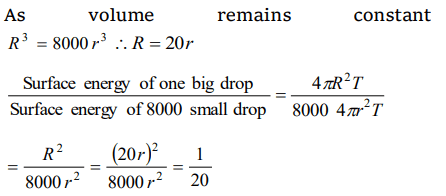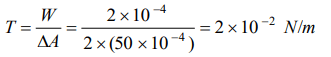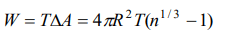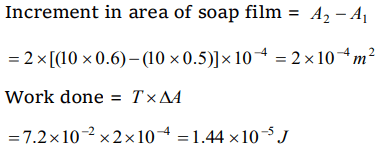## Mechanical Properties of Fluids Questions and Answers Part-8

1. A big drop of radius R is formed by 1000 small droplets of water, then the radius of small drop is
a) R/2
b) R/5
c) R/6
d) R/10

Explanation:2. When $10^{6}$ small drops coalesce to make a new larger drop then the drop
a) Density increases
b) Density decreases
c) Temperature increases
d) Temperature decreases

Explanation: Because energy is liberated

3. Which of the following statements are true in case when two water drops coalesce and make a bigger drop
a) Energy is released
b) Energy is absorbed
c) The surface area of the bigger drop is smaller than the sum of the surface areas of both the drops
d) Both a and c

Explanation: Both a and c

4. 8000 identical water drops are combined to form a big drop. Then the ratio of the final surface energy to the initial surface energy of all the drops together is
a) 1 : 10
b) 1 : 15
c) 1 : 20
d) 1 : 25

Explanation:5. The surface energy of liquid film on a ring of area $0.15m^{2}$ is ( Surface tension of liquid = $5Nm^{-1}$   )
a) 0.75 J
b) 1.5 J
c) 2.25 J
d) 3.0 J

Explanation:6. 8 mercury drops coalesce to form one mercury drop, the energy changes by a factor of
a) 1
b) 2
c) 4
d) 6

Explanation:7. If work done in increasing the size of a soap film from $10 cm\times6 cm$   to $10 cm \times11 cm$   is $2\times 10^{-4}J$   then the surface tension is
a) $2\times 10^{-2}Nm^{-1}$
b) $2\times 10^{-4}Nm^{-1}$
c) $2\times 10^{-6}Nm^{-1}$
d) $2\times 10^{-8}Nm^{-1}$

Explanation:8. A mercury drop of radius 1cm is sprayed into $10^{6}$ drops of equal size. The energy expended in joules is (surface tension of Mercury is $460\times 10^{-3}N\diagup m$   )
a) 0.057
b) 5.7
c) $5.7\times 10^{-4}$
d) $5.7\times 10^{-6}$

Explanation:9. A film of water is formed between two straight parallel wires of length 10cm each separated by 0.5 cm. If their separation is increased by 1 mm while still maintaining their parallelism, how much work will have to be done (Surface tension of water =$7.2\times 10^{-2}N\diagup m$   )
a) $7.22\times 10^{-6}Joule$
b) $1.44\times 10^{-5}Joule$
c) $2.88\times 10^{-5}Joule$
d) $5.76\times 10^{-5}Joule$10. A drop of mercury of radius 2 mm is split into 8 identical droplets. Find the increase in surface energy. (Surface tension of mercury is $0.465 J\diagup m^{2}$   )
a) $23.4\mu J$
b) $18.5\mu J$
c) $26.8\mu J$
d) $16.8\mu J$